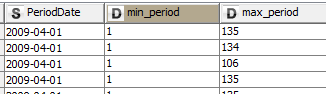# Timestamp data type in R-Snippet

Hi,

I am using R-Snippet to run a long R code.
What I found out is that when you connect a table (knime node) to R-snippet node, in case you have a timestamp, R-Snippet automatically changes it to String data type. Because I need to do calculations in R-Snippet having the variable “perioddate” (format is 2018-06-03) as a timestamp and not as a string, I decided to change the data type of the variable inside the snippet. I tried all the possible commands, which are correct, and non of them is doing the change I need within the R-Snippet.

knime.in\$“PeriodDate2”<- format(as.Date(knime.in\$“PeriodDate”), “%Y-%m-%d”)
library(lubridate)
knime.in\$“PeriodDate2”<-as.Date(parse_date_time(knime.in\$“PeriodDate”,“dmy”))
knime.in\$“PeriodDate2”<-as.Date(knime.in\$“PeriodDate”, “%Y-%m-%d”)

Did anyone had the same problem? did someone found how to solve it?
I would appreciate any feedback, because is important to get the timestamp data type within the R-snippet.

Okay. So, a bit more explanation why I think the timestamp is a problem.
As I said I connect the table with an R-Snippet, I use the knime.in to input the table.
All the data types are correct and the variable “perioddate” has a type as local date.
All good so far. Then I want to use the “perioddate” for a calculation. This calculation has been tested
in R-Studio and works fine. Now, when I run it in R-Snippet I get strange results.

month_sales2 <- data.frame(knime.in %>%
group_by(Customer) %>%
filter(sum(NetSale) > 0) %>%
mutate(
# calculate the first and last date net sales for each customer
sales_in_period = as_date(ifelse(abs(NetSale) > 0, PeriodDate, NA)),
min_period = min(sales_in_period, na.rm = TRUE),
max_period = max(sales_in_period, na.rm = TRUE),
# add one month to max_period
# note: uses the lubricate package
max_period = max_period %m+% months(1)
)%>%

In R-studio, this piece of code created min_period, max_perior and sales_in_period, all having dates.
In R-Snippet, I get some strange values. Eg. for the PeriodDate 2009-04-01, I get min_period 1, max_period 135.

What is wrong with the timestamp in R-Snippet?
I was expecting the R-Snippet to be more straight forward and run exactly the script ans give the same results.

I tried a few things. Bring a String variable to R and then back as a UNIX TimeStamp and convert it back. (have not read your second post yet …)
Edit: maybe you could create a sample workflow with your problem.

knime.in\$date2 <- as.POSIXct(strptime(knime.in\$date1, “%Y-%m-%d”), tz=‘Europe/Berlin’)

kn_example_r_date.knwf (27.6 KB)

Hi,

Can someone help me with the timestamp computation problem I have.
The problem is discribed more clear in my 2nd post.

Thanks.

Maybe you could create a sample Workflow that demonstrates what is happening.

So, I wrote some data manipulations in R. Specifically, I load a .csv file and then I cast the data types.
I have only one timestamp called PeriodDate in this .csv. I cast it as such:

input <- read_csv(“input.csv”, col_types = cols(
PeriodDate = col_date(format = “”),
etc.etc

Then I use the PeriodDate for creating addtional columns, and fitlering. To do these manipulation I use the R-package called lubridate and dplyr. I give you an example of data manipulation below:

month_sales2 <- month_sales1 %>%
group_by(Customer) %>%
filter(sum(NetSale) > 0) %>%
mutate(
sales_in_period = as_date(ifelse(abs(NetSale) > 0, PeriodDate, NA)),
min_period = min(sales_in_period, na.rm = TRUE),
max_period = max(sales_in_period, na.rm = TRUE),
max_period = max_period %m+% months(1)
) %>%

This is the result I am getting in R-studio:Now, I run the R-script in R-Snippet. The PeriodDate is casted as local date. (I tried also to cast it as date and time by using the Date & Time to Legacy Date & Time node), the result in both cases is the same. It seems that R-Snippet does not identify the PeriodDate in the same way, data type, as the R-studio does. Below is piece of code and the result:

month_sales2 <- data.frame(knime.in %>%
group_by(Customer) %>%
filter(sum(NetSale) > 0) %>%
mutate(
sales_in_period = as_date(ifelse(abs(NetSale) > 0, PeriodDate, NA)),
min_period = min(sales_in_period, na.rm = TRUE),
max_period = max(sales_in_period, na.rm = TRUE),
max_period = max_period %m+% months(1)
))
knime.out<-month_sales2How can I make the R-snippet cast the PeriodDate as the R-studio does it?

Hmm maybe not the most creative of all ideas but how about converting the date to a string variable? If you later want to use it in KNIME you might have to convert it back. Another thing could be to extract the UNIX timestamp from the date variable.

If you open the R Snippet and you evaluate the results you could insert something like head(knime.in) to see how your manipulations go and indeed R is displaying the date variables as you would expect.

knime.in\$date2 <- as.POSIXct(strptime(knime.in\$date1, “%Y-%m-%d”), tz=‘Europe/Berlin’)

knime.in\$date3 <- as.character(knime.in\$date2)

kn_example_r_date.knwf (28.3 KB)

Hi mlauber71,

I am not sure if I understand how your proposal solves my problem.

1. solution is to change the R-commands - to handle the PeriodDate as a string, and then compute the min, max etc.
2. how Unix can help me run the current r-script and get the result I need, without changing the r-code?

I was trying to present some options how to deal with TimeVariables. I tried to build a workflow like yours but I am not familiar enough with dplyr. So maybe if you could provide us with a small workflow that produces these unwanted outcome I might have a look at the code within the R Snippet in KNIME.

Until now I did not find a way to bring a date format back to KNIME intact without using either a string or a UNIX Timestamp value.